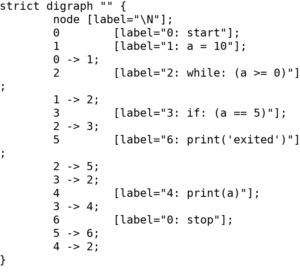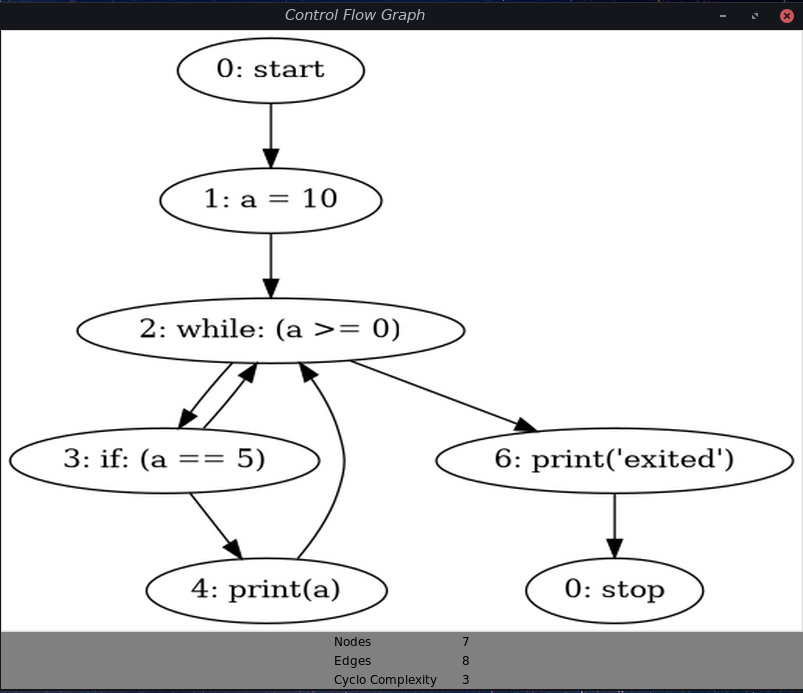Open in App
Not now

# Draw Control Flow Graph using pycfg | Python

• Last Updated : 02 Jan, 2023
Prerequisites: Control Flow Graph, Cyclomatic Complexity

Usually, we draw manual Control Flow Graph using pen and paper by analyzing the control flow of the program. CFG helps us finding independent paths (Cyclomatic Complexity), which leads to the number of test cases required to test the program. We can automate the CFG task using a Python library called pycfg. This library takes a Python script as input and gives graph as output.

We can use pycfg in 2 following ways

## By Directly using the file

2. UnZip it
3. Use `pycfg.py` file

Note: pycfg.py file location after unzipping is `pycfg-0.1/pycfg/pycfg.py`.

Let’s take whiletest.py file to get CFG.

 `a``=` `10``while``(a <``=` `0``):``    ``if` `a ``=``=` `5``:``        ``print``(a)``    ``a ``+``=` `1``print``(``"exited"``)`

Run below command on terminal.

```python path_to/pycfg.py path_to/whiletest.py -d
```

Output:This approach gives output in the form of Graph having Nodes, labeled Sentences, edges between nodes.

## By importing library in the program

With help of importing library and tkinter, we can do way better than just using pycfg.py file alone.

• Representing CFG instead of terminal.
• Find cyclomatic complexity also

Run the below command

```sudo pip install pycfg
```

Once Done, using same whiletest.py for testing. We can run the following python program on whiletest.py.

``` python /path_to/this_file.py /path_to/whiletest.py
```

Below is the code –

 `from` `pycfg.pycfg ``import` `PyCFG, CFGNode, slurp``import` `argparse``import` `tkinter as tk``from` `PIL ``import` `ImageTk, Image`` ` `if` `__name__ ``=``=` `'__main__'``:``    ``parser ``=` `argparse.ArgumentParser()`` ` `    ``parser.add_argument(``'pythonfile'``, ``help` `=``'The python file to be analyzed'``)``    ``args ``=` `parser.parse_args()``    ``arcs ``=` `[]`` ` `    ``cfg ``=` `PyCFG()``    ``cfg.gen_cfg(slurp(args.pythonfile).strip())``    ``g ``=` `CFGNode.to_graph(arcs)``    ``g.draw(args.pythonfile ``+` `'.png'``, prog ``=``'dot'``)`` ` `    ``# Draw using tkinter.``    ``root ``=` `tk.Tk()``    ``root.title(``"Control Flow Graph"``)``    ``img1 ``=` `Image.``open``(``str``(args.pythonfile) ``+` `".png"``)  ``# PIL solution``    ``img1 ``=` `img1.resize((``800``, ``600``), Image.ANTIALIAS)``    ``img ``=` `ImageTk.PhotoImage(img1)``     ` `    ``background ``=``"gray"`` ` `    ``panel ``=` `tk.Label(root, height ``=` `600``, image ``=` `img)``    ``panel.pack(side ``=` `"top"``, fill ``=``"both"``, expand ``=` `"yes"``)``    ``nodes ``=` `g.number_of_nodes()     ``# no. of nodes.``    ``edges ``=` `g.number_of_edges()     ``# no. of Edges.``    ``complexity ``=` `edges ``-` `nodes ``+` `2`         `# Cyclomatic complexity`` ` `    ``frame ``=` `tk.Frame(root, bg ``=` `background)``    ``frame.pack(side ``=``"bottom"``, fill ``=``"both"``, expand ``=` `"yes"``)``         ` `    ``tk.Label(frame, text ``=``"Nodes\t\t"``+``str``(nodes), bg ``=` `background).pack()``    ``tk.Label(frame, text ``=``"Edges\t\t"``+``str``(edges), bg ``=` `background).pack()``    ``tk.Label(frame, text ``=``"Cyclo Complexity\t"``+``             ``str``(complexity), bg ``=` `background).pack()`` ` `    ``root.mainloop()`

Output:Reference: https://pypi.org/project/pycfg/

My Personal Notes arrow_drop_up